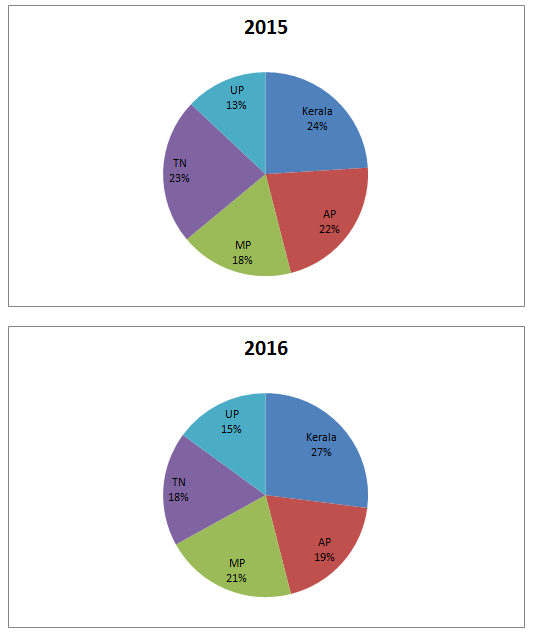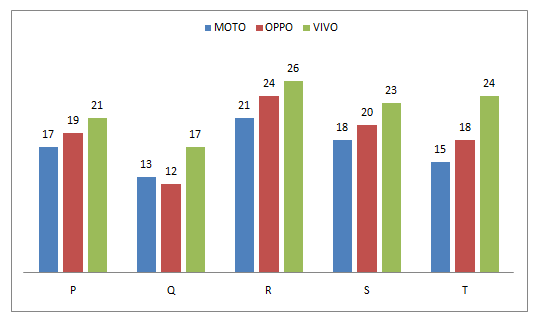# Quantitative Aptitude Questions (Data Interpretation) for SBI Clerk/PO Prelims 2018 Day-96

Dear Readers, SBI is conducting Online Examination for the recruitment of Clerical Cadre and probationary officer. To enrich your preparation here we have providing new series of Data Interpretation – Quantitative Aptitude Questions. Candidates those who are appearing in SBI Clerk/PO Prelims Exams can practice these Quantitative Aptitude average questions daily and make your preparation effective.

[WpProQuiz 2361]

Click “Start Quiz” to attend these Questions and view Solutions

Directions (Q. 1 – 5) Study the following information carefully and answer the given questions:

Following pie chart shows the distribution of total number of readers of a magazine in the year 2015 and 2016 among different states.1. If the total number of readers of the state AP in the year 2015 and 2016 is 57200 and 45600 respectively, then find the difference between the total number of readers of the state Kerala in the year 2015 to that of 2016?
1. 3200
2. 2700
3. 2400
4. 3500
5. None of these
1. If the ratio of total number of readers of the state AP in the year 2016 to that of the state MP in the year 2015 is 4 : 5, then find the ratio of total number of readers of all the given states together in the year 2016 to that in the year 2015?
1. 72 : 95
2. 67 : 88
3. 53 : 92
4. 61 : 76
5. None of these
1. If the total number of readers of all the given states together in the year 2015 and 2016 is 24 lakhs and 28 lakhs respectively, then find the total number of readers of the state MP in the year 2015 and 2016 together?
1. 11.5 lakhs
2. 14.6 lakhs
3. 17.3 lakhs
4. 10.2 lakhs

None of these

1. Total number of readers of state AP in the year 2016 is approximately what percentage of total number of readers of state MP in the year 2015?

(Assume that the total number of readers for both years is same.)

1. 94 %
2. 106 %
3. 88 %
4. 72 %
5. 118 %
1. If total number of readers of state Kerala in the year 2015 is 84000 and the total number of readers of state MP in the year 2016 is 52500, then the ratio of total number of readers of state AP in the year 2015 to that of total number of readers of state Kerala in the year 2016?
1. 157 : 143
2. 181 : 158
3. 165 : 134
4. 154 : 135
5. None of these

Directions (Q. 6 – 10) Study the following information carefully and answer the given questions:

Following bar graph shows the number of mobile users (in thousands) of three brands (i.e., MOTO, OPPO and VIVO) in different cities and the table shows the percentage of males using three brands of mobiles.City MOTO OPPO VIVO Male % Male % Male % P 52 36 48 Q 44 61 32 R 53 46 35 S 36 45 55 T 60 32 36
1. Total number of female mobile users of MOTO and OPPO brands in the city P and T together is approximately what percentage of total number of male mobile users of OPPO and VIVO brands in the city Q and R together?
1. 117 %
2. 125 %
3. 103 %
4. 96 %
5. 88 %
1. Find the ratio between the total female mobile users of MOTO and VIVO brands of city Q and R together to that of total female mobile users of OPPO and VIVO brands of city S and T together?
1. 2897 : 3245
2. 4375 : 3667
3. 4561 : 4895
4. 3243 : 3896
5. None of these
1. Find the difference between the total male mobile users of all the given brands for city P, Q and R together to that of total female mobile users of all the given brands for city R, S and T together?
1. 39870
2. 30690
3. 34560
4. 30890
5. None of these
1. Total number of mobile users of city R and S together is approximately what percentage more/less than the total number of mobile users of city P, Q and T together for all the given brands?
1. 23 % more
2. 27 % less
3. 31 % more
4. 15 % less
5. 25 % more
1. Find the average male mobile users of OPPO brands in all the given cities together?
1. 8154
2. 6578
3. 7992
4. 9156
5. None of these

The total number of readers of the state AP in the year 2015 = 57200

(22/100)*total number of readers in the year 2015 = 57200

Total number of readers in the year 2015 = 57200*(100/22) = 260000

The total number of readers of the state AP in the year 2016 = 45600

(19/100)*total number of readers in the year 2016 = 45600

Total number of readers in the year 2016 = 45600*(100/19) = 240000

Total number of readers of the state Kerala in the year 2015

= > 260000*(24/100) = 62400

Total number of readers of the state Kerala in the year 2016

= > 240000*(27/100) = 64800

Required difference = 64800 – 62400 = 2400

The ratio of total number of readers of the state AP in the year 2016 to that of the state MP in the year 2015 = 4: 5(4x, 5x)

Total number of readers in the year 2016 = 4x*(100/19) = 400x/19

Total number of readers in the year 2015 = 5x*(100/18) = 250x/9

Required ratio = (400x/19): (250x/9) = 72: 95

The total number of readers of all the given states together in the year 2015 is 24 lakhs

The total number of readers of MP in the year 2015 = 24*(18/100) = 4.32 lakhs

The total number of readers of all the given states together in the year 2016 is 28 lakhs

The total number of readers of MP in the year 2016 = 28*(21/100) = 5.88 lakhs

Required sum = 4.32 + 5.88 = 10.2 lakhs

Required % = (19/18)*100 = 105.55 % = 106 %

Total number of readers of state Kerala in the year 2015 = 84000

Total number of readers of state AP in the year 2015

= > 84000*(100/24)*(22/100)

= > 77000

Total number of readers of state MP in the year 2016 = 52500

Total number of readers of state Kerala in the year 2016

= > 52500*(100/21)*(27/100)

= > 67500

Required ratio = 77000: 67500 = 154:135

Direction (6-10)

Total number of female mobile users of MOTO and OPPO brands in the city P and T together

= > [17*(48/100) + 19*(64/100) + 15*(40/100) + 18*(68/100)] thousands

= > [8.16 + 12.16 + 6 + 12.24] thousands

= > 38560

Total number of male mobile users of OPPO and VIVO brands in the city Q and R together

= > [12*(61/100) + 17*(32/100) + 24*(46/100) + 26*(35/100)] thousands

= > [7.32 + 5.44 + 11.04 + 9.10] thousands

= > 32900

Required % = (38560/32900)*100 = 117.2 % = 117 %

The total female mobile users of MOTO and VIVO brands of city Q and R together

= > [13*(56/100) + 17*(68/100) + 21*(47/100) + 26*(65/100)] thousands

= > [7.28 + 11.56 + 9.87 + 16.90] thousands

= > 45610

The total female mobile users of OPPO and VIVO brands of city S and T together

= > [20*(55/100) + 23*(45/100) + 18*(68/100) + 24*(64/100)] thousands

= > [11 + 10.35 + 12.24 + 15.36] thousands

= > 48950

Required ratio = 45610: 48950 = 4561: 4895

The total male mobile users of all the given brands for city P, Q and R together

= > [17*(52/100) + 19*(36/100) + 21*(48/100) + 13*(44/100) + 12*(61/100) + 17*(32/100) + 21*(53/100) + 24*(46/100) + 26*(35/100)] thousands

= > [8.84 + 6.84 + 10.08 + 5.72 + 7.32 + 5.44 + 11.13 + 11.04 + 9.10] thousands

= > 75510

The total female mobile users of all the given brands for city R, S and T together

= > [21*(47/100) + 24*(54/100) + 26*(65/100) + 18*(64/100) + 20*(55/100) + 23*(45/100) + 15*(40/100) + 18*(68/100) + 24*(64/100)] thousands

= > [9.87 + 12.96 + 16.9+ 11.52 + 11 + 10.35 + 6 + 12.24 + 15.36] thousands

= > 106200

Required difference = 106200-75510 = 30690

Total number of mobile users of city R and S together for all the given brands

= > [21 + 24 + 26 + 18 + 20 + 23] thousands

= > 132000

Total number of mobile users of city P, Q and T together for all the given brands

= > [17 + 19 + 21 + 13 + 12 + 17 + 15 + 18 + 24] thousands

= > 156000

Required % = [(156000 – 132000)/156000]*100 = 15.38 % = 15 % less

Total male mobile users of OPPO brands in all the given cities together

= > [19*(36/100) + 12*(61/100) + 24*(46/100) + 20*(45/100) + 18*(32/100)] thousands

= > [6.84 + 7.32 + 11.04 + 9 + 5.76] thousands

= > 39960

Required average = 39960/5 = 7992

Daily Practice Test Schedule | Good Luck

 Topic Daily Publishing Time Daily News Papers & Editorials 8.00 AM Current Affairs Quiz 9.00 AM Quantitative Aptitude “20-20” 11.00 AM Vocabulary (Based on The Hindu) 12.00 PM General Awareness “20-20” 1.00 PM English Language “20-20” 2.00 PM Reasoning Puzzles & Seating 4.00 PM Daily Current Affairs Updates 5.00 PM Data Interpretation / Application Sums (Topic Wise) 6.00 PM Reasoning Ability “20-20” 7.00 PM English Language (New Pattern Questions) 8.00 PM